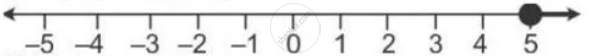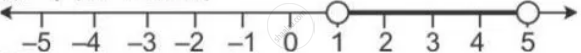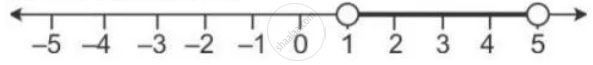Share

P is the Solution Set of 7x – 2 > 4x + 1 and Q is the Solution Set of 9x – 45 ≥ 5(X – 5); Where X ∈ R. Represent: 1) P ∩ Q 2) P – Q 3) P ∩ Q’ on the Different Number of Lines. - ICSE Class 10 - Mathematics

ConceptRepresentation of Solution on the Number Line

Question

P is the solution set of 7x – 2 > 4x + 1 and Q is the solution set of 9x – 45 ≥ 5(x – 5); where x ∈ R. Represent:

1) P ∩ Q

2) P – Q

3) P ∩ Q’

on the different number of lines.

Solution

P = {x : 7x - 2 > 4x + 1, x ∈ R}

7x - 2 > 4x + 1

7x - 4x > 1 + 2

3x > 3

x > 1

and

Q  = {x : 9x - 45 >= 5(x  - 5), x ∈ R}

9x - 45 >=  5x - 25

9x - 5x >= -25 + 45

4x >= 20

x >= 5

1)  P ∩ Q = {x : x >= 5, x ∈ R}2) P - Q = {x : 1 < x < 5, x ∈ R}3) P ∩ Q' = {x : 1 > x < 5, x ∈ R}Is there an error in this question or solution?

APPEARS IN

Solution P is the Solution Set of 7x – 2 > 4x + 1 and Q is the Solution Set of 9x – 45 ≥ 5(X – 5); Where X ∈ R. Represent: 1) P ∩ Q 2) P – Q 3) P ∩ Q’ on the Different Number of Lines. Concept: Representation of Solution on the Number Line.
S